# 【TensorFlow实战笔记】对于TED(en-zh)数据集进行Seq2Seq模型实战，以及对应的Attention机制（tf保存模型读取模型）

## 个人公众号

### AI蜗牛车## 一、前言

environment:

• os: linux
• python: 2.7
• tf: 1.12.0

https://github.com/chehongshu/DL-tenserflow/tree/master/Seq2Seq_Attention

## 二、Se2Seq 模型的基本思想

encoder-decoder模型.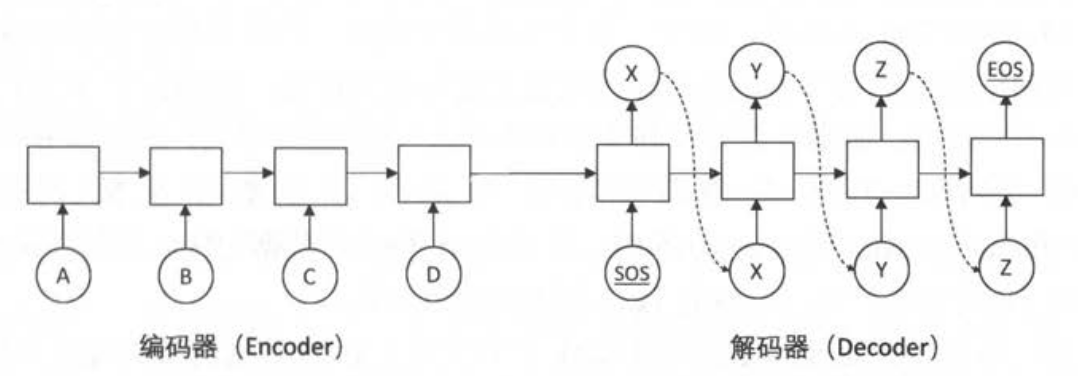从途中可以看到解码器第一个input为SOS,结束为EOS,解释如下。

• SOS Start of sentence
• EOS End of sentence

• 编码器(Encoder)：词向量层（embedding）+rnn，因为没有输出不需要softmax层

• 解码器(Decoder)：输入为单词的词向量 ,输出为 softmax层产生的单词概率,损失函数为 log perplexity，解决器其实就是一个以编码为前提的一个语言模型，所以很多trick和上次讲的LSTM一样可以利用，比如softmax和embedding层可以共享参数。

## 三、下载数据集

https://wit3.fbk.eu/mt.php?release=2015-01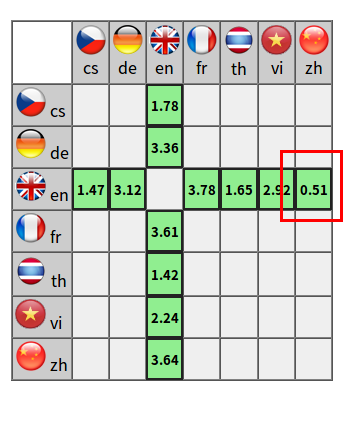## 四、预处理数据

《tensorflow实战笔记》通俗详述RNN理论,LSTM理论,以及LSTM对于PTB数据集进行实战

1. 统计预料中出现的单词，并存到vocab文件中。
2. 为每个单词分配一个单词的id，在这边处理上为行数作为id，这个id就是最后用来转换为数字化的规则。
3. 最后通过这种规则来把文本转换为单词编号形式的文件。

### １．处理原文本格式（切片）

i was studying dl in the ‘60 s,’ 70s.

i was studying dl in the ‘ 60 s , ’ 70s .

https://github.com/moses-smt/mosesdecoder/blob/master/scripts/tokenizer/tokenizer.perl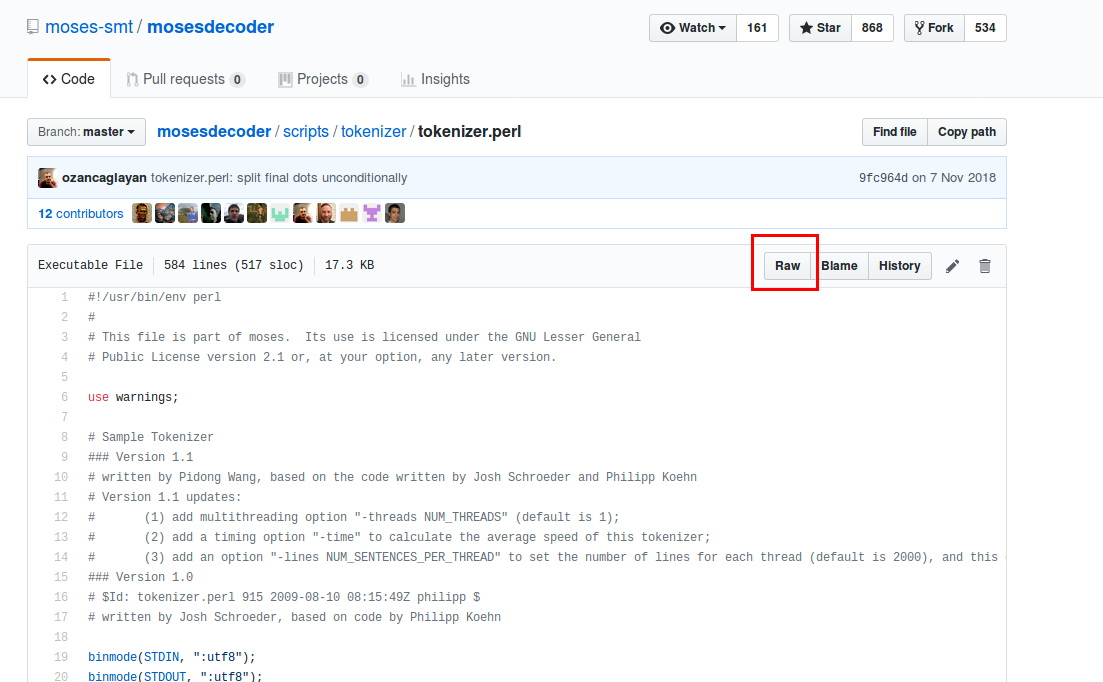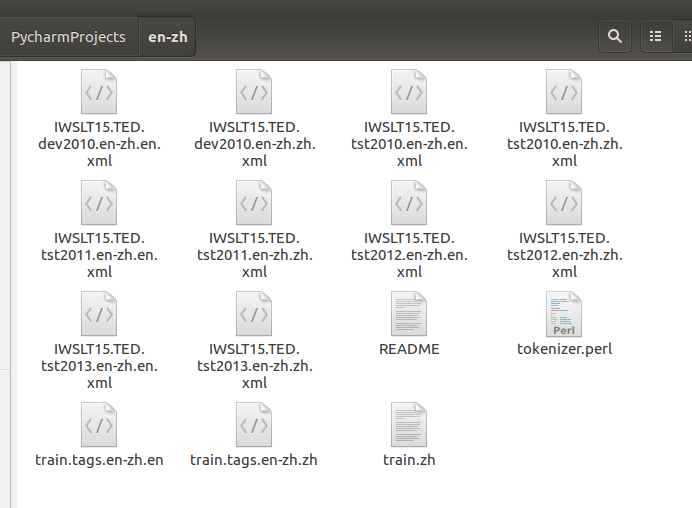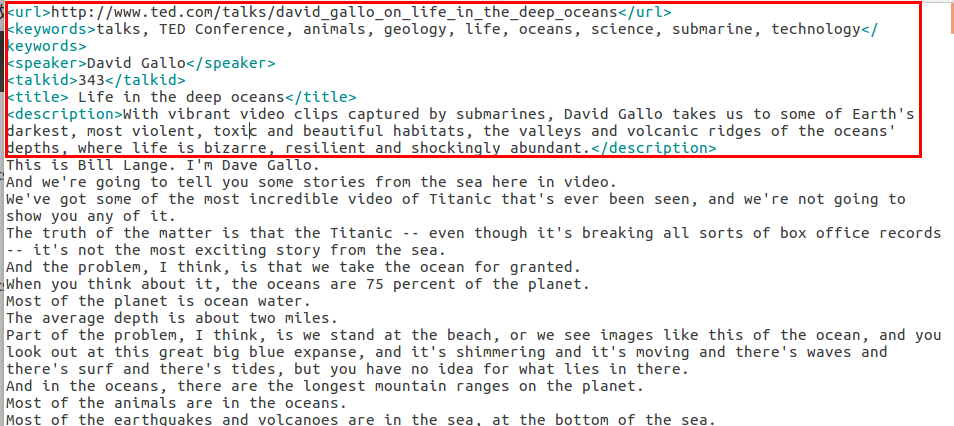# train.raw.en 是原始输入数据,格式为每行一句话: train.txt.en 是输出的文件名。
# -no-escape 参数表示不把标点符号替换为HTML编码(如把引号替换为 ”&quot;”) 。
# -1 en 参数表示输入文件的语言是英文。
perl ./moses_tokenizer.perl -no-escape -1 en < ./train.raw.en > train.txt.en

# train.raw.zh 是原始输入数据,格式为每行一句话: tra 工 n .t xt.zh 是输出的文件名。
# sed ’ s/ I 忡 ’ 表示去除文本中已有的空格。 ’ s/\B/ /g e 将每个字之间的边界替换为空格。
sed ’ s/ //g; s/\B/ /g ’ . / train . raw .zh > train.txt.zh


https://github.com/chehongshu/DL-tenserflow/tree/master/Seq2Seq_Attention/en-zh

### ２．处理原文本格式（切片）

generate_VOCAB.py

# -*- coding:UTF-8 -*-

"""
@Author:Che_Hongshu
@Modify:2019.1.8
"""
import codecs
import collections
from operator import itemgetter

DATA_TYPE = "english"  # 将DATA_TYPE先后设置为chinese,english得到中英文ＶＯＣＡＢ文件

if DATA_TYPE == "chinese":  # 翻译语料的中文部分
RAW_DATA = "./en-zh/train.txt.zh"
VOCAB_OUTPUT = "zh.vocab"
VOCAB_SIZE = 4000  #中文词汇表单词个数
elif DATA_TYPE == "english":  # 翻译语料的英文部分
RAW_DATA = "./en-zh/train.txt.en"
VOCAB_OUTPUT = "en.vocab"
VOCAB_SIZE = 10000  #英文词汇表单词个数

counter = collections.Counter() #一个计数器，统计每个单词出现的次数

with codecs.open(RAW_DATA, "r", "utf-8") as f: #utf-8格式读取
for line in f:
for word in line.strip().split(): #line.strip().split()相当于把每一行的前后空格去掉，再根据空格分词生成list
counter[word] += 1 #统计相同单词出现次数＋１
#  Counter 集成于 dict 类，因此也可以使用字典的方法，此类返回一个以元素为 key 、元素个数为 value 的 Counter 对象集合
#　依据key排序　itermgetter(1)为降序
sorted_word_to_cnt = sorted(counter.items(), key=itemgetter(1), reverse=True)

#  转换成单词string的list
sorted_words_list = [x for x in sorted_word_to_cnt]

#  加入句子结束符
sorted_words_list = ["<unk>", "<sos>", "<eos>"] + sorted_words_list

if len(sorted_words_list) > VOCAB_SIZE:
sorted_words_list = sorted_words_list[:VOCAB_SIZE]

with codecs.open(VOCAB_OUTPUT, 'w', 'utf-8') as file_output:
for word in sorted_words_list:
file_output.write(word + '\n')



# -*- coding:UTF-8 -*-

"""
@Author:Che_Hongshu
@Modify:2018.1.8
"""
import codecs

DATA_TYPE = "chinese"  # 将DATA_TYPE先后设置为chinese,english得到中英文ＶＯＣＡＢ文件

if DATA_TYPE == "chinese":  # 翻译语料的中文部分
RAW_DATA = "./en-zh/train.txt.zh"
VOCAB = "zh.vocab"
OUTPUT_DATA = "train.zh"
elif DATA_TYPE == "english":  # 翻译语料的英文部分
RAW_DATA = "./en-zh/train.txt.en"
VOCAB = "en.vocab"
OUTPUT_DATA = "train.en"

with codecs.open(VOCAB, 'r', 'utf-8') as f_vocab:  #打开文件进入读操作
vocab = [w.strip() for w in f_vocab.readlines()]  # 先把所有词转换成list
# 把每个词和所在行数对应起来并且zip打包成(“词”，行数)格式转换成dict格式
word_to_id = {k: v for (k, v) in zip(vocab, range(len(vocab)))}

# 返回id 如果在词汇表文件中则返回对应的id即可，如果没有则返回'<unk>'
def get_id(word):
return word_to_id[word] if word in word_to_id else word_to_id['<unk>']

# 打开文件
fin = codecs.open(RAW_DATA, 'r', 'utf-8')
fout = codecs.open(OUTPUT_DATA, 'w', 'utf-8')

for line in fin:
words = line.strip().split() + ["<eos>"] #每一行的单词变成sring list格式，每一句话后面加上一个结束符号
out_line = ' '.join([str(get_id(w)) for w in words]) + '\n' #这一行中的每个单词取出对应的id之后用空格相连接
fout.write(out_line)

# 关闭文件
fin.close()
fout.close()


example：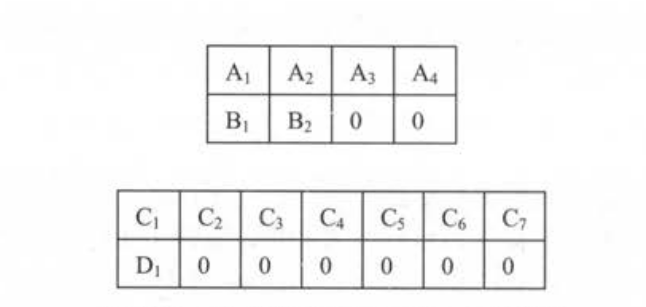但是TensorFlow提供了 tf.nn.dynamic_rnn函数来很方便的实现这一功能，解决这个问题。dynamic_rnn 对每一个batch的数据读取两个输入。
①输入数据的内容（维度为[batch_size, time]）
②输入数据的长度（维度为[time]）
并且使用dynamic_rnn时每个batch的最大序列长度不需要相同。例如上面的例子，batch大小为２，第一个batch的维度是2x4，而第二个batch的维度是2x7。在训练中dynamic_rnn会根据每个batch的最大长度动态展开到需要的层数，其实就是对每个batch本身的最大长度没有关系，函数会自动动态(dynamic)调整
• 第二，在设计损失函数时需要特别将填充位置的损失权重设置为 0 ,这样在填充位
置产生的预测不会影响梯度的计算。

# -*- coding:UTF-8 -*-

import tensorflow as tf

MAX_LEN = 50                        # 限定句子的最大单词数量
SOS_ID = 1                          # 目标语言词汇表中<sos>的ID

"""
function: 数据batching,产生最后输入数据格式
Parameters:
file_path-数据路径
Returns:
dataset-　每个句子－对应的长度组成的TextLineDataset类的数据集对应的张量
CSDN:
http://blog.csdn.net/qq_33431368
"""
# 使用Dataset从一个文件中读取一个语言的数据。
# 数据的格式为每一句话，单词已经转化为单词编号
def MakeDataset(file_path):
dataset = tf.data.TextLineDataset(file_path)

# map(function, sequence[, sequence, ...]) -> list
# 通过定义可以看到，这个函数的第一个参数是一个函数，剩下的参数是一个或多个序列，返回值是一个集合。
# function可以理解为是一个一对一或多对一函数，map的作用是以参数序列中的每一个元素调用function函数，返回包含每次function函数返回值的list。
# lambda argument_list: expression
# 其中lambda是Python预留的关键字,argument_list和expression由用户自定义
# argument_list参数列表, expression 为函数表达式
# 根据空格将单词编号切分开并放入一个一维向量
dataset = dataset.map(lambda string: tf.string_split([string]).values)
# 将字符串形式的单词编号转化为整数
dataset = dataset.map(lambda string: tf.string_to_number(string, tf.int32))
# 统计每个句子的单词数量，并与句子内容一起放入Dataset
dataset = dataset.map(lambda x: (x, tf.size(x)))
return dataset

"""
function: 从源语言文件src_path和目标语言文件trg_path中分别读取数据，并进行填充和batching操作
Parameters:
src_path-源语言，即被翻译的语言,英语.
trg_path-目标语言，翻译之后的语言,汉语.
batch_size-batch的大小
Returns:
dataset-　每个句子－对应的长度　组成的TextLineDataset类的数据集
CSDN:
http://blog.csdn.net/qq_33431368
"""
def MakeSrcTrgDataset(src_path, trg_path, batch_size):
# 首先分别读取源语言数据和目标语言数据
src_data = MakeDataset(src_path)
trg_data = MakeDataset(trg_path)
# 通过zip操作将两个Dataset合并为一个Dataset，现在每个Dataset中每一项数据ds由4个张量组成
# ds是源句子
# ds是源句子长度
# ds是目标句子
# ds是目标句子长度
#https://blog.csdn.net/qq_32458499/article/details/78856530这篇博客看一下可以细致了解一下Dataset这个库，以及.map和.zip的用法
dataset = tf.data.Dataset.zip((src_data, trg_data))

# 删除内容为空(只包含<eos>)的句子和长度过长的句子
def FilterLength(src_tuple, trg_tuple):
((src_input, src_len), (trg_label, trg_len)) = (src_tuple, trg_tuple)
# tf.logical_and 相当于集合中的and做法，后面两个都为true最终结果才会为true，否则为false
# tf.greater Returns the truth value of (x > y),所以以下所说的是句子长度必须得大于一也就是不能为空的句子
# tf.less_equal Returns the truth value of (x <= y),所以所说的是长度要小于最长长度
src_len_ok = tf.logical_and(tf.greater(src_len, 1), tf.less_equal(src_len, MAX_LEN))
trg_len_ok = tf.logical_and(tf.greater(trg_len, 1), tf.less_equal(trg_len, MAX_LEN))
return tf.logical_and(src_len_ok, trg_len_ok) #两个都满足才返回true

# filter接收一个函数Func并将该函数作用于dataset的每个元素，根据返回值True或False保留或丢弃该元素，True保留该元素，False丢弃该元素
# 最后得到的就是去掉空句子和过长的句子的数据集
dataset = dataset.filter(FilterLength)

# 解码器需要两种格式的目标句子：
# 1.解码器的输入(trg_input), 形式如同'<sos> X Y Z'
# 2.解码器的目标输出(trg_label), 形式如同'X Y Z <eos>'
# 上面从文件中读到的目标句子是'X Y Z <eos>'的形式，我们需要从中生成'<sos> X Y Z'形式并加入到Dataset
# 编码器只有输入,没有输出,而解码器有输入也有输出，输入为<sos>＋(除去最后一位eos的label列表)
# 例如train.en最后都为2,ｉｄ为２就是eos
def MakeTrgInput(src_tuple, trg_tuple):
((src_input, src_len), (trg_label, trg_len)) = (src_tuple, trg_tuple)
# tf.concat用法 https://blog.csdn.net/qq_33431368/article/details/79429295
trg_input = tf.concat([[SOS_ID], trg_label[:-1]], axis=0)
return ((src_input, src_len), (trg_input, trg_label, trg_len))
dataset = dataset.map(MakeTrgInput)

# 随机打乱训练数据
dataset = dataset.shuffle(10000)

# 规定填充后的输出的数据维度
(tf.TensorShape([None]),    # 源句子是长度未知的向量
tf.TensorShape([])),       # 源句子长度是单个数字
(tf.TensorShape([None]),    # 目标句子(解码器输入)是长度未知的向量
tf.TensorShape([None]),    # 目标句子(解码器目标输出)是长度未知的向量
tf.TensorShape([]))        # 目标句子长度(输出)是单个数字
)

return batched_dataset


## 五、seq2seq模型建立

### 1．train_model

《tensorflow实战笔记》通俗详述RNN理论,LSTM理论,以及LSTM对于PTB数据集进行实战一样也使用一个双层
LSTM 作为循环神经网络的主体,并在 Softmax 层和词向量层之间共享参数，增加如下：

• 增加了一个循环神经网络作为编码器（如前面示意图）
• 使用 Dataset 动态读取数据，而不是直接将所有数据读入内存（这个就是Dataset输入数据的特点）
• 每个 batch 完全独立，不需要在batch之间传递状态（因为不是一个文件整条句子，每个句子之间没有传递关系）
• 每训练200步便将模型参数保存到一个 checkpoint 中，以后用于测试。
"""
function: seq2seq模型
Parameters:
Returns:
CSDN:
http://blog.csdn.net/qq_33431368
"""
class NMTModel(object):
"""
function: 模型初始化
Parameters:
Returns:
CSDN:
http://blog.csdn.net/qq_33431368
"""
def __init__(self):

# 定义编码器和解码器所使用的LSTM结构
self.enc_cell = tf.nn.rnn_cell.MultiRNNCell(
[tf.nn.rnn_cell.LSTMCell(HIDDEN_SIZE) for _ in range(NUM_LAYERS)])
self.dec_cell = tf.nn.rnn_cell.MultiRNNCell(
[tf.nn.rnn_cell.LSTMCell(HIDDEN_SIZE) for _ in range(NUM_LAYERS)])
# 为源语言和目标语言分别定义词向量
self.src_embedding = tf.get_variable('src_emb', [SRC_VOCAB_SIZE, HIDDEN_SIZE])
self.trg_embedding = tf.get_variable('trg_emb', [TRG_VOCAB_SIZE, HIDDEN_SIZE])
# 定义softmax层的变量
if SHARE_EMB_AND_SOFTMAX:
self.softmax_weight = tf.transpose(self.trg_embedding)
else:
self.softmax_weight = tf.get_variable('weight', [HIDDEN_SIZE, TRG_VOCAB_SIZE])
self.softmax_bias = tf.get_variable('softmax_loss', [TRG_VOCAB_SIZE])

"""
function: 在forward函数中定义模型的前向计算图
Parameters:
MakeSrcTrgDataset函数产生的五种张量如下（全部为张量）
src_input: 编码器输入（源数据）
src_size : 输入大小
trg_input：解码器输入（目标数据）
trg_label：解码器输出（目标数据）
trg_size：　输出大小
Returns:
cost_per_token: cost操作op
train_op: 训练操作op
CSDN:
http://blog.csdn.net/qq_33431368
"""
def forward(self, src_input, src_size, trg_input, trg_label, trg_size):
batch_size = tf.shape(src_input)
# 将输入和输出单词转为词向量（rnn中输入数据都要转换成词向量）
# 相当于input中的每个id对应的embedding中的向量转换
src_emb = tf.nn.embedding_lookup(self.src_embedding, src_input)
trg_emb = tf.nn.embedding_lookup(self.trg_embedding, trg_input)
# 在词向量上进行dropout
src_emb = tf.nn.dropout(src_emb, KEEP_PROB)
trg_emb = tf.nn.dropout(trg_emb, KEEP_PROB)
# 使用dynamic_rnn构造编码器
# 编码器读取源句子每个位置的词向量，输出最后一步的隐藏状态enc_state
# 因为编码器是一个双层LSTM，因此enc_state是一个包含两个LSTMStateTuple类的tuple，
# 每个LSTMStateTuple对应编码器中一层的状态
# enc_outputs是顶层LSTM在每一步的输出，它的维度是[batch_size, max_time, HIDDEN_SIZE]
# seq2seq模型中不需要用到enc_outputs,而attention模型会用到它
with tf.variable_scope('encoder'):
enc_outputs, enc_state = tf.nn.dynamic_rnn(self.enc_cell, src_emb, src_size, dtype=tf.float32)
# 使用dynamic_rnn构造解码器
# 解码器读取目标句子每个位置的词向量，输出的dec_outputs为每一步顶层LSTM的输出
# dec_outputs的维度是[batch_size, max_time, HIDDEN_SIZE]
# initial_state=enc_state表示用编码器的输出来初始化第一步的隐藏状态
# 编码器最后编码结束最后的状态为解码器初始化的状态
with tf.variable_scope('decoder'):
dec_outputs, _ = tf.nn.dynamic_rnn(self.dec_cell, trg_emb, trg_size, initial_state=enc_state)
# 计算解码器每一步的log perplexity
#　输出重新转换成shape为[,HIDDEN_SIZE]
output = tf.reshape(dec_outputs, [-1, HIDDEN_SIZE])
#  计算解码器每一步的softmax概率值
logits = tf.matmul(output, self.softmax_weight) + self.softmax_bias
#  交叉熵损失函数，算loss
loss = tf.nn.sparse_softmax_cross_entropy_with_logits(labels=tf.reshape(trg_label, [-1]), logits=logits)
# 在计算平均损失时，需要将填充位置的权重设置为0，以避免无效位置的预测干扰模型的训练
label_weights = tf.reshape(label_weights, [-1])
cost = tf.reduce_sum(loss * label_weights)
cost_per_token = cost / tf.reduce_sum(label_weights)
# 定义反向传播操作
trainable_variables = tf.trainable_variables()
# 控制梯度大小，定义优化方法和训练步骤
# 算出每个需要更新的值的梯度，并对其进行控制
# 利用梯度下降优化算法进行优化.学习率为1.0
return cost_per_token, train_op


### 2．全部训练代码

# -*- coding:UTF-8 -*-
"""
@Author:Che_Hongshu
@Modify:2018.1.9
"""
import tensorflow as tf

SRC_TRAIN_DATA = 'train.en'  # 源语言输入文件
TRG_TRAIN_DATA = 'train.zh'  # 目标语言输入文件
CHECKPOINT_PATH = './model/seq2seq_ckpt'  # checkpoint保存路径
HIDDEN_SIZE = 1024                  # LSTM的隐藏层规模
NUM_LAYERS = 2                      # 深层循环神经网络中LSTM结构的层数
SRC_VOCAB_SIZE = 10000              # 源语言词汇表大小
TRG_VOCAB_SIZE = 4000               # 目标语言词汇表大小
BATCH_SIZE = 100                    # 训练数据batch的大小
NUM_EPOCH = 5                       # 使用训练数据的轮数
KEEP_PROB = 0.8                     # 节点不被dropout的概率
SHARE_EMB_AND_SOFTMAX = True        # 在softmax层和词向量层之间共享参数
MAX_LEN = 50                        # 限定句子的最大单词数量
SOS_ID = 1                          # 目标语言词汇表中<sos>的ID

"""
function: 数据batching,产生最后输入数据格式
Parameters:
file_path-数据路径
Returns:
dataset-　每个句子－对应的长度组成的TextLineDataset类的数据集对应的张量
CSDN:
http://blog.csdn.net/qq_33431368
"""
def MakeDataset(file_path):
dataset = tf.data.TextLineDataset(file_path)

# map(function, sequence[, sequence, ...]) -> list
# 通过定义可以看到，这个函数的第一个参数是一个函数，剩下的参数是一个或多个序列，返回值是一个集合。
# function可以理解为是一个一对一或多对一函数，map的作用是以参数序列中的每一个元素调用function函数，返回包含每次function函数返回值的list。
# lambda argument_list: expression
# 其中lambda是Python预留的关键字,argument_list和expression由用户自定义
# argument_list参数列表, expression 为函数表达式
# 根据空格将单词编号切分开并放入一个一维向量
dataset = dataset.map(lambda string: tf.string_split([string]).values)
# 将字符串形式的单词编号转化为整数
dataset = dataset.map(lambda string: tf.string_to_number(string, tf.int32))
# 统计每个句子的单词数量，并与句子内容一起放入Dataset
dataset = dataset.map(lambda x: (x, tf.size(x)))
return dataset

"""
function: 从源语言文件src_path和目标语言文件trg_path中分别读取数据，并进行填充和batching操作
Parameters:
src_path-源语言，即被翻译的语言,英语.
trg_path-目标语言，翻译之后的语言,汉语.
batch_size-batch的大小
Returns:
dataset-　每个句子－对应的长度　组成的TextLineDataset类的数据集
CSDN:
http://blog.csdn.net/qq_33431368
"""
def MakeSrcTrgDataset(src_path, trg_path, batch_size):
# 首先分别读取源语言数据和目标语言数据
src_data = MakeDataset(src_path)
trg_data = MakeDataset(trg_path)
# 通过zip操作将两个Dataset合并为一个Dataset，现在每个Dataset中每一项数据ds由4个张量组成
# ds是源句子
# ds是源句子长度
# ds是目标句子
# ds是目标句子长度
#https://blog.csdn.net/qq_32458499/article/details/78856530这篇博客看一下可以细致了解一下Dataset这个库，以及.map和.zip的用法
dataset = tf.data.Dataset.zip((src_data, trg_data))

# 删除内容为空(只包含<eos>)的句子和长度过长的句子
def FilterLength(src_tuple, trg_tuple):
((src_input, src_len), (trg_label, trg_len)) = (src_tuple, trg_tuple)
# tf.logical_and 相当于集合中的and做法，后面两个都为true最终结果才会为true，否则为false
# tf.greater Returns the truth value of (x > y),所以以下所说的是句子长度必须得大于一也就是不能为空的句子
# tf.less_equal Returns the truth value of (x <= y),所以所说的是长度要小于最长长度
src_len_ok = tf.logical_and(tf.greater(src_len, 1), tf.less_equal(src_len, MAX_LEN))
trg_len_ok = tf.logical_and(tf.greater(trg_len, 1), tf.less_equal(trg_len, MAX_LEN))
return tf.logical_and(src_len_ok, trg_len_ok) #两个都满足才返回true

# filter接收一个函数Func并将该函数作用于dataset的每个元素，根据返回值True或False保留或丢弃该元素，True保留该元素，False丢弃该元素
# 最后得到的就是去掉空句子和过长的句子的数据集
dataset = dataset.filter(FilterLength)

# 解码器需要两种格式的目标句子：
# 1.解码器的输入(trg_input), 形式如同'<sos> X Y Z'
# 2.解码器的目标输出(trg_label), 形式如同'X Y Z <eos>'
# 上面从文件中读到的目标句子是'X Y Z <eos>'的形式，我们需要从中生成'<sos> X Y Z'形式并加入到Dataset
# 编码器只有输入,没有输出,而解码器有输入也有输出，输入为<sos>＋(除去最后一位eos的label列表)
# 例如train.en最后都为2,ｉｄ为２就是eos
def MakeTrgInput(src_tuple, trg_tuple):
((src_input, src_len), (trg_label, trg_len)) = (src_tuple, trg_tuple)
# tf.concat用法 https://blog.csdn.net/qq_33431368/article/details/79429295
trg_input = tf.concat([[SOS_ID], trg_label[:-1]], axis=0)
return ((src_input, src_len), (trg_input, trg_label, trg_len))
dataset = dataset.map(MakeTrgInput)

# 随机打乱训练数据
dataset = dataset.shuffle(10000)

# 规定填充后的输出的数据维度
(tf.TensorShape([None]),    # 源句子是长度未知的向量
tf.TensorShape([])),       # 源句子长度是单个数字
(tf.TensorShape([None]),    # 目标句子(解码器输入)是长度未知的向量
tf.TensorShape([None]),    # 目标句子(解码器目标输出)是长度未知的向量
tf.TensorShape([]))        # 目标句子长度(输出)是单个数字
)

return batched_dataset

"""
function: seq2seq模型
Parameters:
Returns:
CSDN:
http://blog.csdn.net/qq_33431368
"""
class NMTModel(object):
"""
function: 模型初始化
Parameters:
Returns:
CSDN:
http://blog.csdn.net/qq_33431368
"""
def __init__(self):

# 定义编码器和解码器所使用的LSTM结构
self.enc_cell = tf.nn.rnn_cell.MultiRNNCell(
[tf.nn.rnn_cell.LSTMCell(HIDDEN_SIZE) for _ in range(NUM_LAYERS)])
self.dec_cell = tf.nn.rnn_cell.MultiRNNCell(
[tf.nn.rnn_cell.LSTMCell(HIDDEN_SIZE) for _ in range(NUM_LAYERS)])
# 为源语言和目标语言分别定义词向量
self.src_embedding = tf.get_variable('src_emb', [SRC_VOCAB_SIZE, HIDDEN_SIZE])
self.trg_embedding = tf.get_variable('trg_emb', [TRG_VOCAB_SIZE, HIDDEN_SIZE])
# 定义softmax层的变量
if SHARE_EMB_AND_SOFTMAX:
self.softmax_weight = tf.transpose(self.trg_embedding)
else:
self.softmax_weight = tf.get_variable('weight', [HIDDEN_SIZE, TRG_VOCAB_SIZE])
self.softmax_bias = tf.get_variable('softmax_loss', [TRG_VOCAB_SIZE])

"""
function: 在forward函数中定义模型的前向计算图
Parameters:
MakeSrcTrgDataset函数产生的五种张量如下（全部为张量）
src_input: 编码器输入（源数据）
src_size : 输入大小
trg_input：解码器输入（目标数据）
trg_label：解码器输出（目标数据）
trg_size：　输出大小
Returns:
CSDN:
http://blog.csdn.net/qq_33431368
"""
def forward(self, src_input, src_size, trg_input, trg_label, trg_size):
batch_size = tf.shape(src_input)
# 将输入和输出单词转为词向量（rnn中输入数据都要转换成词向量）
# 相当于input中的每个id对应的embedding中的向量转换
src_emb = tf.nn.embedding_lookup(self.src_embedding, src_input)
trg_emb = tf.nn.embedding_lookup(self.trg_embedding, trg_input)
# 在词向量上进行dropout
src_emb = tf.nn.dropout(src_emb, KEEP_PROB)
trg_emb = tf.nn.dropout(trg_emb, KEEP_PROB)
# 使用dynamic_rnn构造编码器
# 编码器读取源句子每个位置的词向量，输出最后一步的隐藏状态enc_state
# 因为编码器是一个双层LSTM，因此enc_state是一个包含两个LSTMStateTuple类的tuple，
# 每个LSTMStateTuple对应编码器中一层的状态
# enc_outputs是顶层LSTM在每一步的输出，它的维度是[batch_size, max_time, HIDDEN_SIZE]
# seq2seq模型中不需要用到enc_outputs,而attention模型会用到它
with tf.variable_scope('encoder'):
enc_outputs, enc_state = tf.nn.dynamic_rnn(self.enc_cell, src_emb, src_size, dtype=tf.float32)
# 使用dynamic_rnn构造解码器
# 解码器读取目标句子每个位置的词向量，输出的dec_outputs为每一步顶层LSTM的输出
# dec_outputs的维度是[batch_size, max_time, HIDDEN_SIZE]
# initial_state=enc_state表示用编码器的输出来初始化第一步的隐藏状态
# 编码器最后编码结束最后的状态为解码器初始化的状态
with tf.variable_scope('decoder'):
dec_outputs, _ = tf.nn.dynamic_rnn(self.dec_cell, trg_emb, trg_size, initial_state=enc_state)
# 计算解码器每一步的log perplexity
#　输出重新转换成shape为[,HIDDEN_SIZE]
output = tf.reshape(dec_outputs, [-1, HIDDEN_SIZE])
#  计算解码器每一步的softmax概率值
logits = tf.matmul(output, self.softmax_weight) + self.softmax_bias
#  交叉熵损失函数，算loss
loss = tf.nn.sparse_softmax_cross_entropy_with_logits(labels=tf.reshape(trg_label, [-1]), logits=logits)
# 在计算平均损失时，需要将填充位置的权重设置为0，以避免无效位置的预测干扰模型的训练
label_weights = tf.reshape(label_weights, [-1])
cost = tf.reduce_sum(loss * label_weights)
cost_per_token = cost / tf.reduce_sum(label_weights)
# 定义反向传播操作
trainable_variables = tf.trainable_variables()
# 控制梯度大小，定义优化方法和训练步骤
# 算出每个需要更新的值的梯度，并对其进行控制
# 利用梯度下降优化算法进行优化.学习率为1.0
return cost_per_token, train_op

"""
function: 使用给定的模型model上训练一个epoch，并返回全局步数，每训练200步便保存一个checkpoint
Parameters:
session :  会议
cost_op :  计算loss的操作op
train_op：　训练的操作op
saver：　　保存model的类
step：　　　训练步数
Returns:
CSDN:
http://blog.csdn.net/qq_33431368
"""
def run_epoch(session, cost_op, train_op, saver, step):
# 训练一个epoch
# 重复训练步骤直至遍历完Dataset中所有数据
while True:
try:
# 运行train_op并计算cost_op的结果也就是损失值，训练数据在main()函数中以Dataset方式提供
cost, _ = session.run([cost_op, train_op])
# 步数为１０的倍数进行打印
if step % 10 == 0:
print('After %d steps, per token cost is %.3f' % (step, cost))
# 每200步保存一个checkpoint
if step % 200 == 0:
saver.save(session, CHECKPOINT_PATH, global_step=step)
step += 1
except tf.errors.OutOfRangeError:
break
return step

"""
function: 主函数
Parameters:
Returns:
CSDN:
http://blog.csdn.net/qq_33431368
"""
def main():
# 定义初始化函数
initializer = tf.random_uniform_initializer(-0.05, 0.05)
# 定义训练用的循环神经网络模型
with tf.variable_scope('nmt_model', reuse=None, initializer=initializer):
train_model = NMTModel()
# 定义输入数据
data = MakeSrcTrgDataset(SRC_TRAIN_DATA, TRG_TRAIN_DATA, BATCH_SIZE)
iterator = data.make_initializable_iterator()
(src, src_size), (trg_input, trg_label, trg_size) = iterator.get_next()
# 定义前向计算图，输入数据以张量形式提供给forward函数
cost_op, train_op = train_model.forward(src, src_size, trg_input, trg_label, trg_size)
# 训练模型
# 保存模型
saver = tf.train.Saver()
step = 0
with tf.Session() as sess:
# 初始化全部变量
tf.global_variables_initializer().run()
# 进行NUM_EPOCH轮数
for i in range(NUM_EPOCH):
print('In iteration: %d' % (i + 1))
sess.run(iterator.initializer)
step = run_epoch(sess, cost_op, train_op, saver, step)

if __name__ == '__main__':
main()


### 3．全部测试代码

# -*- coding:UTF-8 -*-

"""
@Author:Che_Hongshu
@Modify:2018.1.10
"""
import tensorflow as tf
import codecs
import sys

# 读取checkpoint的路径。4800表示是训练程序在第4800步保存的checkpoint
CHECKPOINT_PATH = 'model/seq2seq_ckpt-9000'

# 模型参数。必须与训练时的模型参数保持一致
HIDDEN_SIZE = 1024              # LSTM的隐藏层规模
NUM_LAYERS = 2                  # 深层循环神经网络中LSTM结构的层数
SRC_VOCAB_SIZE = 10000          # 源语言词汇表大小
TRG_VOCAB_SIZE = 4000           # 目标语言词汇表大小
SHARE_EMB_AND_SOFTMAX = True    # 在softmax层和词向量层之间共享参数

# 词汇表文件
SRC_VOCAB = "en.vocab"
TRG_VOCAB = "zh.vocab"

# 词汇表中<sos>和<eos>的ID，在解码过程中需要用<sos>作为第一步的输入，<eos>为最终的输出结果，因此需要知道这两个符号的ID
SOS_ID = 1
EOS_ID = 2

"""
function: seq2seq模型
Parameters:
Returns:
CSDN:
http://blog.csdn.net/qq_33431368
"""
class NMTModel(object):
# 在模型的初始化函数中定义模型要用到的变量
def __init__(self):
# 定义编码器和解码器所使用的LSTM结构
self.enc_cell = tf.nn.rnn_cell.MultiRNNCell(
[tf.nn.rnn_cell.LSTMCell(HIDDEN_SIZE) for _ in range(NUM_LAYERS)])
self.dec_cell = tf.nn.rnn_cell.MultiRNNCell(
[tf.nn.rnn_cell.LSTMCell(HIDDEN_SIZE) for _ in range(NUM_LAYERS)])
# 为源语言和目标语言分别定义词向量
self.src_embedding = tf.get_variable('src_emb', [SRC_VOCAB_SIZE, HIDDEN_SIZE])
self.trg_embedding = tf.get_variable('trg_emb', [TRG_VOCAB_SIZE, HIDDEN_SIZE])
# 定义softmax层的变量
if SHARE_EMB_AND_SOFTMAX:
self.softmax_weight = tf.transpose(self.trg_embedding)
else:
self.softmax_weight = tf.get_variable('weight', [HIDDEN_SIZE, TRG_VOCAB_SIZE])
self.softmax_bias = tf.get_variable('softmax_loss', [TRG_VOCAB_SIZE])

"""
function: 利用模型进行推理
Parameters:
src_input: 输入句子,在这里就是已经转换成id文本的英文句子。
Returns:
CSDN:
http://blog.csdn.net/qq_33431368
"""
def inference(self, src_input):
# 虽然输入只有一个句子，但因为dynamic_rnn要求输入时batch的形式，因此这里将输入句子整理为大小为1的batch
src_size = tf.convert_to_tensor([len(src_input)], dtype=tf.int32)
src_input = tf.convert_to_tensor([src_input], dtype=tf.int32)
src_emb = tf.nn.embedding_lookup(self.src_embedding, src_input)

# 使用dynamic_rnn 构造编码器
with tf.variable_scope('encoder'):
enc_outputs, enc_state = tf.nn.dynamic_rnn(self.enc_cell, src_emb, dtype=tf.float32)
# 设置解码的最大步数 避免在极端情况出现无限循环的问题
MAX_DEC_LEN = 100
with tf.variable_scope('decoder/rnn/multi_rnn_cell'):
# 使用一个变长的TensorArray来存储生成的句子
init_array = tf.TensorArray(dtype=tf.int32, size=0, dynamic_size=True, clear_after_read=False)
# 填入第一个单词<sos>作为解码器的输入
init_array = init_array.write(0, SOS_ID)
# 构建初始的循环状态，循环状态包含循环神经网络的隐藏状态，保存生成句子TensorArray, 以及记录解码步数的一个整数step
init_loop_var = (enc_state, init_array, 0)
"""
function: tf.while_loop的循环条件
Parameters:
state:　隐藏状态
trg_ids:　目标句子的id的集合，也就是上面定义的 TensorArray
step:　解码步数
Returns:
解码器没有输出< eos > , 或者没有达到最大步数则输出True,循环继续。
CSDN:
http://blog.csdn.net/qq_33431368
"""
def contunue_loop_condition(state, trg_ids, step):
tf.less(step, MAX_DEC_LEN-1))

"""
function: tf.while_loop的循环条件
Parameters:
state:　隐藏状态
trg_ids:　目标句子的id的集合，也就是上面定义的 TensorArray
step:　解码步数
Returns:
next_state:　下一个隐藏状态
trg_ids: 新的得到的目标句子
step+1:　下一步
CSDN:
http://blog.csdn.net/qq_33431368
"""
def loop_body(state, trg_ids, step):
# 读取最后一步输出的单词，并读取其词向量,作为下一步的输入
trg_emb = tf.nn.embedding_lookup(self.trg_embedding, trg_input)
# 这里不使用dynamic_rnn,而是直接调用dec_cell向前计算一步
# 每个RNNCell都有一个call方法，使用方式是：(output, next_state) = call(input, state)。
# 每调用一次RNNCell的call方法，就相当于在时间上“推进了一步”，这就是RNNCell的基本功能。
dec_outputs, next_state = self.dec_cell.call(inputs=trg_emb, state=state)
# 计算每个可能的输出单词对应的logit,并选取logit值最大的单词作为这一步的输出
# 解码器输出经过softmax层，算出每个结果的概率取最大为最终输出
output = tf.reshape(dec_outputs, [-1, HIDDEN_SIZE])
logits = (tf.matmul(output, self.softmax_weight) + self.softmax_bias)
# tf.argmax(logits, axis=1, output_type=tf.int32)相当于一维里的数据概率返回醉倒的索引值，即比step小一个
next_id = tf.argmax(logits, axis=1, output_type=tf.int32)
# 将这部输出的单词写入循环状态的trg_ids中，也就是继续写入到结果里
trg_ids = trg_ids.write(step+1, next_id)
return next_state, trg_ids, step+1
# 执行tf.while_loop,返回最终状态
# while_loop(contunue_loop_condition, loop_body, init_loop_var)
# contunue_loop_condition 循环条件
# loop_body 循环体
#　循环的起始状态，所以循环条件和循环体的输入参数就是起始状态
state, trg_ids, step = tf.while_loop(contunue_loop_condition, loop_body, init_loop_var)
# 将TensorArray中元素叠起来当做一个Tensor输出
return trg_ids.stack()

def main():

# 定义训练用的循环神经网络模型。
with tf.variable_scope("nmt_model", reuse=None):
model = NMTModel()

# 定义个测试句子。
test_en_text = "The sea is blue . <eos>"
print(test_en_text)

# 根据英文词汇表，将测试句子转为单词ID。
with codecs.open(SRC_VOCAB, "r", "utf-8") as vocab:
src_vocab = [w.strip() for w in vocab.readlines()]
# 运用dict,　将单词和id对应起来组成字典，用于后面的转换。
src_id_dict = dict((src_vocab[x], x) for x in range(SRC_VOCAB_SIZE))
test_en_ids = [(src_id_dict[en_text] if en_text in src_id_dict else src_id_dict['<unk>'])
for en_text in test_en_text.split()]
print(test_en_ids)

# 建立解码所需的计算图。
output_op = model.inference(test_en_ids)
sess = tf.Session()
saver = tf.train.Saver()
saver.restore(sess, CHECKPOINT_PATH)

# 读取翻译结果。
output_ids = sess.run(output_op)
print(output_ids)

# 根据中文词汇表，将翻译结果转换为中文文字。
with codecs.open(TRG_VOCAB, "r", "utf-8") as f_vocab:
trg_vocab = [w.strip() for w in f_vocab.readlines()]
output_text = ''.join([trg_vocab[x] for x in output_ids])

# 输出翻译结果。 utf-8编码
print(output_text.encode('utf8'))
sess.close()

if __name__ == '__main__':
main()


## 六、Attention机制（注意力机制）

### 1.先看Attention理论

https://blog.csdn.net/wuzqchom/article/details/75792501
https://blog.csdn.net/guohao_zhang/article/details/79540014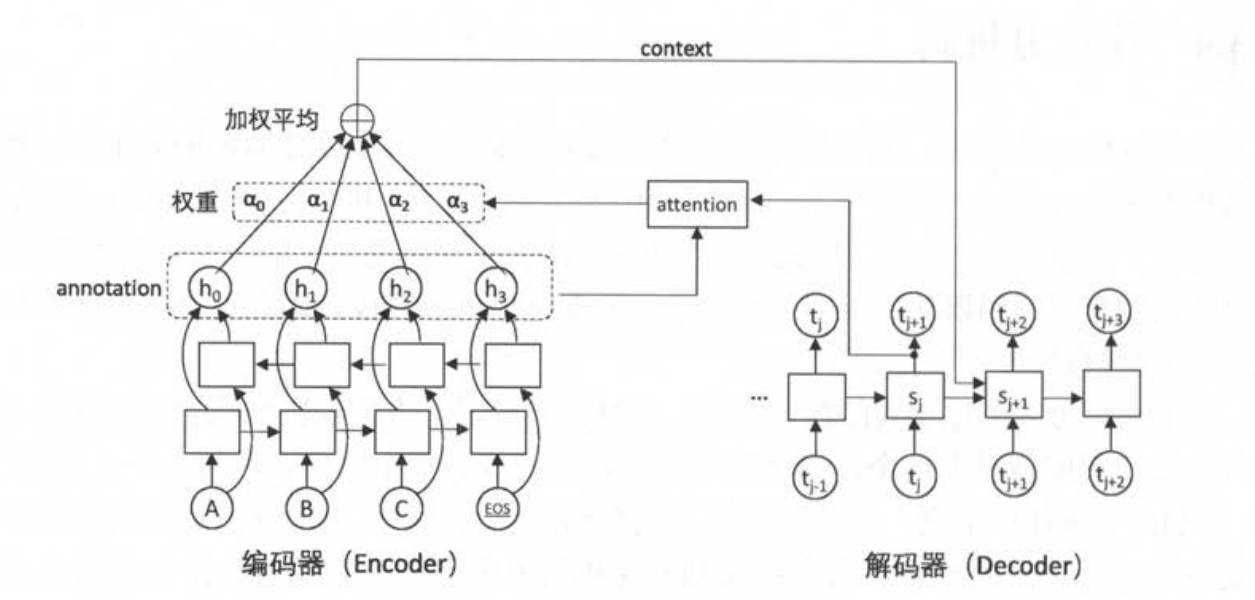• 编码器从单向的LSTM结构，更改为双向的LSTM结构，所以编码器的输出也随之变为两个方向一同的LSTM合成输出
• 需要根据编码器输出来计算Attention权重（有相应的计算模型）
• 解码器和Attention机制封装成一个更为高级的循环神经网络结构

### 2.把NMTmodel替换成NMTmodel_Attention

#### train中需要替代的代码如下：

"""
function: seq2seq模型－Attention机制
Parameters:
Returns:
CSDN:
http://blog.csdn.net/qq_33431368
"""
# attention 编码器双向循环，解码器单向循环
class NMTModel_Attention(object):
# 在模型的初始化函数中定义模型要用到的变量
def __init__(self):
# 定义编码器和解码器所使用的LSTM结构
self.enc_cell_fw = tf.nn.rnn_cell.LSTMCell(HIDDEN_SIZE)  #前向
self.enc_cell_bw = tf.nn.rnn_cell.LSTMCell(HIDDEN_SIZE)  #反向

self.dec_cell = tf.nn.rnn_cell.MultiRNNCell(
[tf.nn.rnn_cell.LSTMCell(HIDDEN_SIZE) for _ in range(NUM_LAYERS)])
# 为源语言和目标语言分别定义词向量
self.src_embedding = tf.get_variable('src_emb', [SRC_VOCAB_SIZE, HIDDEN_SIZE])
self.trg_embedding = tf.get_variable('trg_emb', [TRG_VOCAB_SIZE, HIDDEN_SIZE])
# 定义softmax层的变量
# 只有解码器需要用到softmax
if SHARE_EMB_AND_SOFTMAX:
self.softmax_weight = tf.transpose(self.trg_embedding)
else:
self.softmax_weight = tf.get_variable('weight', [HIDDEN_SIZE, TRG_VOCAB_SIZE])
self.softmax_bias = tf.get_variable('softmax_loss', [TRG_VOCAB_SIZE])

"""
function: 在forward函数中定义模型的前向计算图
Parameters:
MakeSrcTrgDataset函数产生的五种张量如下（全部为张量）
src_input: 编码器输入（源数据）
src_size : 输入大小
trg_input：解码器输入（目标数据）
trg_label：解码器输出（目标数据）
trg_size：　输出大小
Returns:
CSDN:
http://blog.csdn.net/qq_33431368
"""
def forward(self, src_input, src_size, trg_input, trg_label, trg_size):
batch_size = tf.shape(src_input)
# 将输入和输出单词转为词向量
src_emb = tf.nn.embedding_lookup(self.src_embedding, src_input)
trg_emb = tf.nn.embedding_lookup(self.trg_embedding, trg_input)
# 在词向量上进行dropout
src_emb = tf.nn.dropout(src_emb, KEEP_PROB)
trg_emb = tf.nn.dropout(trg_emb, KEEP_PROB)
# 编码器
with tf.variable_scope('encoder'):
# 构造编码器时，使用birdirectional_dynamic_rnn构造双向循环网络。
# 双向循环网络的顶层输出enc_outputs是一个包含两个张量的tuple，每个张量的
# 维度都是[batch_size, max_time, HIDDEN_SIZE],代表两个LSTM在每一步的输出
enc_outputs, enc_state = tf.nn.bidirectional_dynamic_rnn(self.enc_cell_fw, self.enc_cell_bw, src_emb,
src_size, dtype=tf.float32)
# 将两个LSTM输出拼接为一个张量
enc_outputs = tf.concat([enc_outputs, enc_outputs], -1)

# 使用dynamic_rnn构造解码器
with tf.variable_scope('decoder'):
# 选择注意力权重的计算模型。BahdanauAttention是使用一个隐藏层的前馈神经网络
# memory_sequence_length是一个维度为[batch_size]的张量，代表batch中每个句子的长度
# Attention需要根据这个信息把填充位置的注意里权重设置为0
attention_mechanism = tf.contrib.seq2seq.BahdanauAttention(HIDDEN_SIZE, enc_outputs,
memory_sequence_length=src_size)
# 将解码器的循环神经网络self.dec_cell和注意力一起封装成更高层的循环神经网络
attention_cell = tf.contrib.seq2seq.AttentionWrapper(self.dec_cell, attention_mechanism,
attention_layer_size=HIDDEN_SIZE)
# 使用attention_cell和dynamic_rnn构造编码器
# 这里没有指定init_state,也就是没有使用编码器的输出来初始化输入，而完全依赖注意力作为信息来源
dec_outputs, _ = tf.nn.dynamic_rnn(attention_cell, trg_emb, trg_size, dtype=tf.float32)

# 计算解码器每一步的log perplexity
output = tf.reshape(dec_outputs, [-1, HIDDEN_SIZE])
logits = tf.matmul(output, self.softmax_weight) + self.softmax_bias
loss = tf.nn.sparse_softmax_cross_entropy_with_logits(labels=tf.reshape(trg_label, [-1]), logits=logits)
# 在计算平均损失时，需要将填充位置的权重设置为0，以避免无效位置的预测干扰模型的训练
label_weights = tf.reshape(label_weights, [-1])
cost = tf.reduce_sum(loss * label_weights)
cost_per_token = cost / tf.reduce_sum(label_weights)
# 定义反向传播操作
trainable_variables = tf.trainable_variables()
# 控制梯度大小，定义优化方法和训练步骤
return cost_per_token, train_op


#### decoding需要替代的代码

"""
function: seq2seq模型－Attention
Parameters:
Returns:
CSDN:
http://blog.csdn.net/qq_33431368
"""
class NMTModel_Attention(object):
# 在模型的初始化函数中定义模型要用到的变量
def __init__(self):
# 定义编码器和解码器所使用的LSTM结构
self.enc_cell_fw = tf.nn.rnn_cell.LSTMCell(HIDDEN_SIZE)
self.enc_cell_bw = tf.nn.rnn_cell.LSTMCell(HIDDEN_SIZE)
self.dec_cell = tf.nn.rnn_cell.MultiRNNCell(
[tf.nn.rnn_cell.LSTMCell(HIDDEN_SIZE) for _ in range(NUM_LAYERS)])
# 为源语言和目标语言分别定义词向量
self.src_embedding = tf.get_variable('src_emb', [SRC_VOCAB_SIZE, HIDDEN_SIZE])
self.trg_embedding = tf.get_variable('trg_emb', [TRG_VOCAB_SIZE, HIDDEN_SIZE])
# 定义softmax层的变量
if SHARE_EMB_AND_SOFTMAX:
self.softmax_weight = tf.transpose(self.trg_embedding)
else:
self.softmax_weight = tf.get_variable('weight', [HIDDEN_SIZE, TRG_VOCAB_SIZE])
self.softmax_bias = tf.get_variable('softmax_loss', [TRG_VOCAB_SIZE])

"""
function: 利用模型进行推理
Parameters:
src_input: 输入句子,在这里就是已经转换成id文本的英文句子。
Returns:
CSDN:
http://blog.csdn.net/qq_33431368
"""
def inference(self, src_input):
# 虽然输入只有一个句子，但因为dynamic_rnn要求输入时batch的形式，因此这里将输入句子整理为大小为1的batch
src_size = tf.convert_to_tensor([len(src_input)], dtype=tf.int32)
src_input = tf.convert_to_tensor([src_input], dtype=tf.int32)
src_emb = tf.nn.embedding_lookup(self.src_embedding, src_input)

# 使用bidirectional_dynamic_rnn 构造编码器（双向LSTM）
with tf.variable_scope('encoder'):
enc_outputs, enc_state = tf.nn.bidirectional_dynamic_rnn(
self.enc_cell_fw, self.enc_cell_bw, src_emb, src_size, dtype=tf.float32)
# 将两个LSTM输出拼接为一个张量
enc_outputs = tf.concat([enc_outputs, enc_outputs], -1)
with tf.variable_scope("decoder"):
# 定义解码器使用的注意力机制。
# 选择注意力权重的计算模型。BahdanauAttention是使用一个隐藏层的前馈神经网络
# memory_sequence_length是一个维度为[batch_size]的张量，代表batch中每个句子的长度
# Attention需要根据这个信息把填充位置的注意里权重设置为0
attention_mechanism = tf.contrib.seq2seq.BahdanauAttention(
HIDDEN_SIZE, enc_outputs,
memory_sequence_length=src_size)

# 将解码器的循环神经网络self.dec_cell和注意力一起封装成更高层的循环神经网络。
attention_cell = tf.contrib.seq2seq.AttentionWrapper(
self.dec_cell, attention_mechanism,
attention_layer_size=HIDDEN_SIZE)

# 设置解码的最大步数 避免在极端情况出现无限循环的问题
MAX_DEC_LEN = 100
with tf.variable_scope('decoder/rnn/attention_wrapper'):
# 使用一个变长的TensorArray来存储生成的句子
init_array = tf.TensorArray(dtype=tf.int32, size=0, dynamic_size=True, clear_after_read=False)
# 填入第一个单词<sos>作为解码器的输入
init_array = init_array.write(0, SOS_ID)
# 构建初始的循环状态，循环状态包含循环神经网络的隐藏状态，保存生成句子TensorArray, 以及记录解码步数的一个整数step
init_loop_var = (attention_cell.zero_state(batch_size=1, dtype=tf.float32), init_array, 0)
# tf.while_loop的循环条件
"""
function: tf.while_loop的循环条件
Parameters:
state:　隐藏状态
trg_ids:　目标句子的id的集合，也就是上面定义的 TensorArray
step:　解码步数
Returns:
解码器没有输出< eos > , 或者没有达到最大步数则输出True,循环继续。
CSDN:
http://blog.csdn.net/qq_33431368
"""
def contunue_loop_condition(state, trg_ids, step):
tf.less(step, MAX_DEC_LEN-1))

"""
function: tf.while_loop的循环条件
Parameters:
state:　隐藏状态
trg_ids:　目标句子的id的集合，也就是上面定义的 TensorArray
step:　解码步数
Returns:
next_state:　下一个隐藏状态
trg_ids: 新的得到的目标句子
step+1:　下一步
CSDN:
http://blog.csdn.net/qq_33431368
"""
def loop_body(state, trg_ids, step):
# 读取最后一步输出的单词，并读取其词向量
trg_emb = tf.nn.embedding_lookup(self.trg_embedding, trg_input)
# 这里不使用dynamic_rnn,而是直接调用call向前计算一步
# 每个RNNCell都有一个call方法，使用方式是：(output, next_state) = call(input, state)。
# 每调用一次RNNCell的call方法，就相当于在时间上“推进了一步”，这就是RNNCell的基本功能。
dec_outputs, next_state = attention_cell.call(inputs=trg_emb, state=state)
# 计算每个可能的输出单词对应的logit,并选取logit值最大的单词作为这一步的输出
# 解码器输出经过softmax层，算出每个结果的概率取最大为最终输出
output = tf.reshape(dec_outputs, [-1, HIDDEN_SIZE])
logits = (tf.matmul(output, self.softmax_weight) + self.softmax_bias)
# tf.argmax(logits, axis=1, output_type=tf.int32)相当于一维里的数据概率返回醉倒的索引值，即比step小一个
next_id = tf.argmax(logits, axis=1, output_type=tf.int32)
# 将这部输出的单词写入循环状态的trg_ids中
trg_ids = trg_ids.write(step+1, next_id)
return next_state, trg_ids, step+1
# while_loop(contunue_loop_condition, loop_body, init_loop_var)
# contunue_loop_condition 循环条件
# loop_body 循环体
# 　循环的起始状态，所以循环条件和循环体的输入参数就是起始状态
state, trg_ids, step = tf.while_loop(contunue_loop_condition, loop_body, init_loop_var)
# 将TensorArray中元素叠起来当做一个Tensor输出
return trg_ids.stack()


## 七、测试结果（以下为Attention_model训练测试结果图）

１．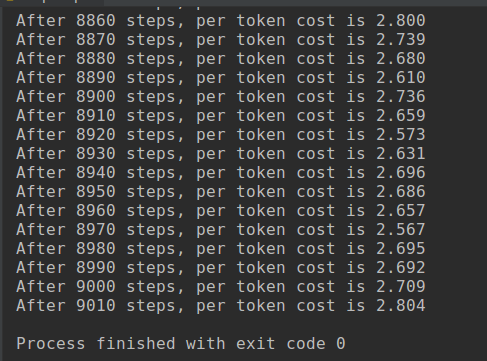２．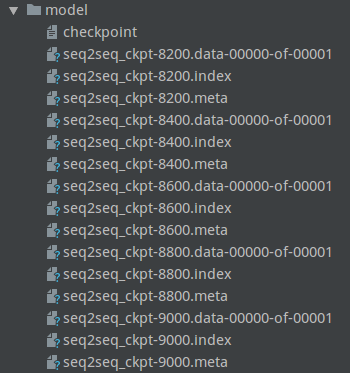３．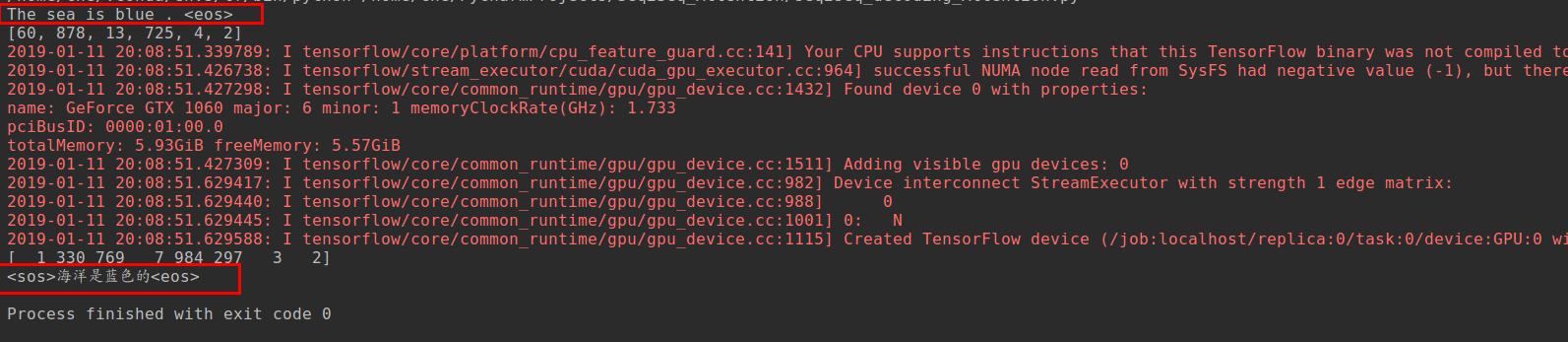## 八、github代码地址(欢迎star, fork)

### github代码地址：

https://github.com/chehongshu/DL-tenserflow/tree/master/Seq2Seq_Attention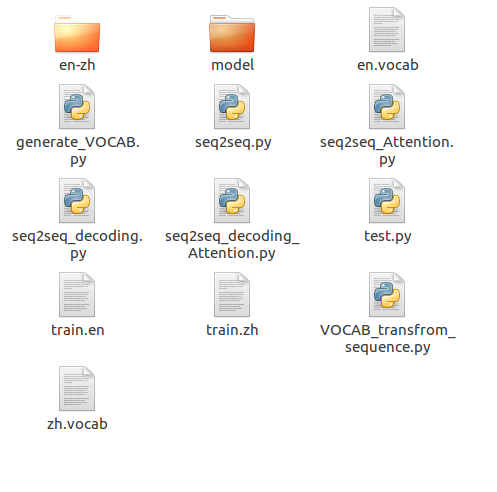PS： 如果觉得本篇本章对您有所帮助，欢迎关注、评论、点赞！Github给个Star就更完美了_！

## 欢迎关注本人公众号## Reference

https://www.w3cschool.cn/tensorflow_python/tensorflow_python-8pwb2dbr.html

http://www.runoob.com/python/python-func-map.html

https://blog.csdn.net/zjuxsl/article/details/79437563

https://blog.csdn.net/lvjc2010/article/details/78777098/

https://blog.csdn.net/chixujohnny/article/details/51025336

https://blog.csdn.net/qq_32458499/article/details/78856530

https://blog.csdn.net/qq_16234613/article/details/81703228

https://blog.csdn.net/weixin_31767897/article/details/79365968

https://blog.csdn.net/guolindonggld/article/details/79256018

11-23
05-17994405-05285
09-28236
01-24732
05-102062
09-231万+
06-051450
01-232642
©️2020 CSDN 皮肤主题: Age of Ai 设计师:meimeiellie